# BACK TO COLLEGE: A RUBY POSTFIX REPL CALCULATOR

By: Saurav

2017-08-30 20:12:00 UTC

Normally for me its always so awesome to work on full stack consumer based projects. Working on a project which changes people’s lives or adds value and you can see your work getting appreciated is always the best feeling for a developer. But amidst all the fancy stuffs and combinations you posses. And all those moments you feel confident in your abilities about solving any problem, sometimes you gotta take a break and go back and train the basics again. You know! the simple jabs and the crosses we were taught in the college days.

Its been more than an year since I completed my grad studies but there was something about solving those assignments and figuring out simple stuffs within time constraints of an hour or so. I miss it. Recently, I bumped into this old school assignment problem by LSU CS department and I was like okay! lets see what I would do different than what I used to do in grad school. Especially where in college, I was never taught the importance of Behavior/Test Driven Development, it was just an after thought. Given the course load, I don’t blame anyone but now I know how important it is for developer happiness and saving time in testing.

Problem Statement: Design a REPL(Read Eval Print Loop) Reverse Polish notation / postfix command line calculator. When q or CTRL+D or CTRL+C is pressed, give a nice exit from the command line. The calculator should perform the basic “+”, “-”, “/”, “*” operations. Design it in a way to make it easy to change, easy to maintain and easy to expand to any other app

Lets get started! Shall we?Step 1. The first thing I did was to research through post fix notation and refresh the data structures course I took in school. Choosing best suited DS for this task solves a lot of issues. Lets think about it.

When you give a calculator two operands and one operator, it will give you the output. For e.g. 4 + 5 will give 9. In postfix expression, it will be 4 5 + = 9. Complexity arises when we do successive operations. For e.g. 6 5 2–4 + * = (whats your answer?).

Here we have successive operations to be done as the user is typing the input. i.e. we need a data structure to store “the last step’s result”. Rings a bell? LAST IN FIRST OUT! voila! Theoretically, that’s a Stack. But just for experimentation, I will do the operations with queue as well to see what’s best suited for the job by practical trial and error as well, just to prove a point

Step 2. The next step would be to setup the project and write tests and use them to help us write the initial code and test it. I will use Rspec(A great resource is provided by relishapp, go check it out) for testing just for the ease and my comfort level with it. Create a Gem file in a folder and install Rspec within. Run Bundle and let it do the magic of package managements.

```Gemfile
source ‘http://rubygems.org'
gem ‘rspec-rails’, ‘~> 3.5```

Finally, bootstrap Rspec using:

`rails generate rspec:install`

Step 3. Lets write our first test and let it guide us to our goal of creating our first version of the project.
In the spec folder generated, create a new file: calculator_spec.rb and write the first test.

```describe "Calculator" do
context 'initialize' do
it "initializes the class calculator" do
calculator = Calculator.new
expect(calculator).to be_an_instance_of(Calculator)
end
end
end```

Lets run our tests and see what happens: rspec spec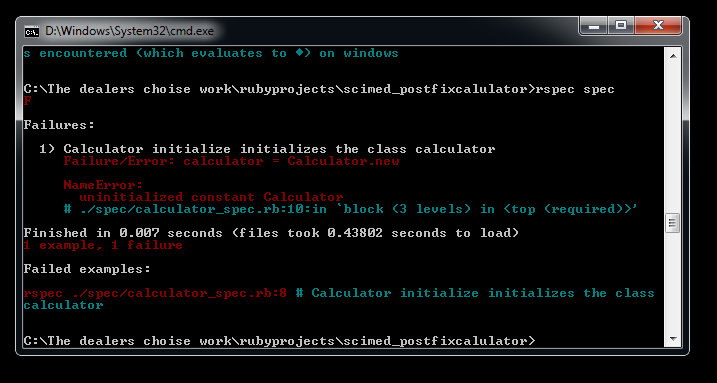```NameError:
uninitialized constant Calculator```

That means there is no such calculator class as such known which we are making instance of. Lets create a class Calculator in a lib folder and make it available to rspec through:

```class Calculator
end
require ‘calculator.rb’```

Lets run out tests again! : Rspec spec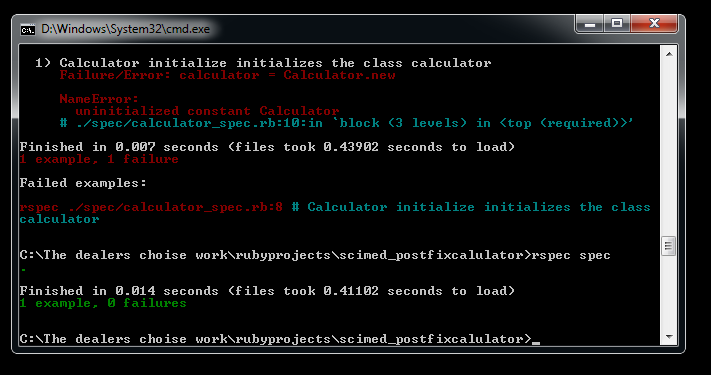Step 4. Now that we have been shown the light to walk towards, lets go ahead and use initializer method to have our initial data structure within it. We can write a test for this as well but I normally escape testing on things which are obvious.

```class Calculator
def initialize
# Will use array as stack
@stack = Array.new
# A total which will be our output to print
@total = 0.0
end
end```

Step 5. Now, lets write the main tests the calculator need to pass in order to calculate.

```#These all will be inside describe “Calculator” do end block
context 'calculate' do
it "calculates the input: test case 1" do
calculator = Calculator.new
result = calculator.calculate('4 5 +')
expect(result).to eq 9.0
end
end```

Lets run our test: rspec spec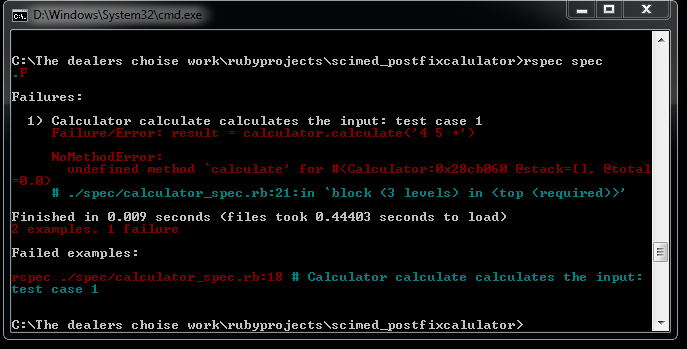```NoMethodError:
undefined method 'calculate' for #<Calculator>```

which means, we need a method name calculate inside the class which will do calculations. This method takes an input and will return total (result):

```class Calculator

def initialize
# Will use array as stack
@stack = Array.new
# A total which will be our output to print
@total = 0.0
end
def calculate(input)
return @total
end
end```

Lets run the test again!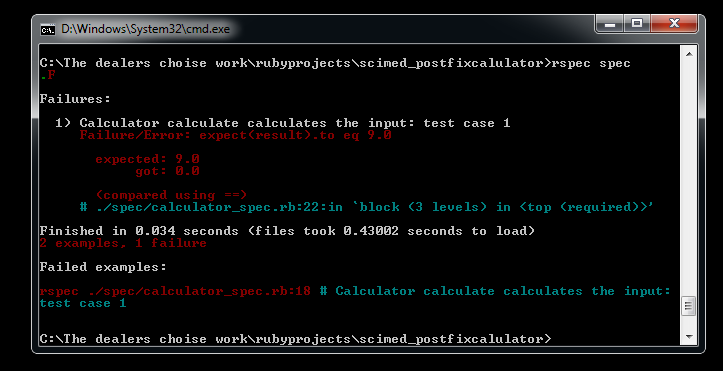Good! we solved the last problem but the new problem is:

```expected : 9.0
got: nil```

Step 6. Now, we need the logic here to actually calculate and pass the tests. Just for the sake of learning lets use a queue and develop the logic and see what happens and understand why its so important to write tests holistically and invest time in it.

```Lets think of the algorithm:
a. A user will give an input which will be a string.
b. The string should be broken down checking for space in between.
c. For each character in a loop, we will see what type it is.
If it an operator, we will do operation by popping last two  operands and doing the operation storing the result in the total and    putting the result back in the DS.
If not, we will update the total to the operand and put it in the DS
d. Finally, we will print out the total after the loop
e. For error cases, for q, and CTRL+D, we will handle it gracefully```

Here is a basic code I wrote according to the logic

```class Calculator
def initialize
@stack = Queue.new
@total = 0.0
end
def calculate(input)
input_to_array = input.split(' ')
input_to_array.each do |i|
if i == "q" or i == "\u0004"
@stack.clear
@total = 0.0
p "bye bye!"
return
end
if i == '+'

@first = @stack.pop.to_f
@second = @stack.pop.to_f
@total = @first + @second
@stack << @total

elsif i == '-'

@first = @stack.pop.to_f
@second = @stack.pop.to_f
@total = @first - @second
@stack << @total
elsif i == '/'

@first = @stack.pop.to_f
@second = @stack.pop.to_f
@total = @first / @second
@stack << @total

elsif i == '*'

@first = @stack.pop.to_f
@second = @stack.pop.to_f
@total = @first * @second
@stack << @total

else
@total = i.to_f
@stack << @total
end

end
return @total
end
end```

Now, lets run out tests and see if it passes!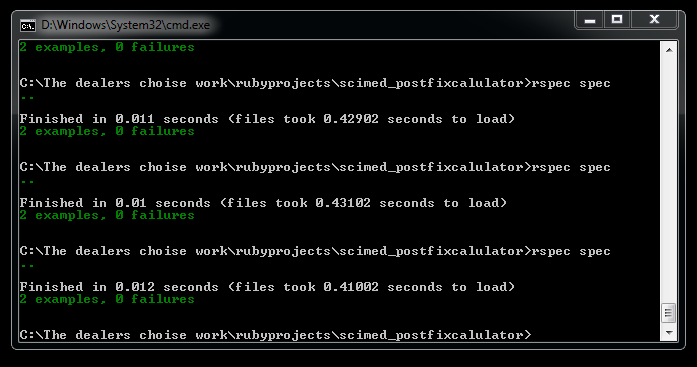And there you have it! We got a calculator running and passing our tests. Lets add input sanitization and checks and make this code good to handle error cases as well.

```class Calculator
def initialize
@stack = Queue.new
@total = 0.0
end
def calculate(input)
input_to_array = input.split(' ')
input_to_array.each do |i|
if i == "q" or i == "\u0004"
@stack.clear
@total = 0.0
p "bye bye!"
return
end
# input sanitization and check for legit input, if input is not legit, calculator should raise error.
if !!(i =~ /\A\d|\+|\-|\*|\/\z/)
if i == '+'
# p "addition"
@first = @stack.pop.to_f
@second = @stack.pop.to_f
@total = @first + @second
@stack << @total

elsif i == '-'
# p "subtraction"
@first = @stack.pop.to_f
@second = @stack.pop.to_f
@total = @first - @second
@stack << @total
elsif i == '/'
# p "division"
@first = @stack.pop.to_f
@second = @stack.pop.to_f
@total = @first / @second
@stack << @total
elsif i == '*'
# p "multiplication"
@first = @stack.pop.to_f
@second = @stack.pop.to_f
@total = @first * @second
@stack << @total

else
# p i
@total = i.to_f
@stack << @total
end
else
p "invalid input recognized, please check your input string"
return "invalid input recognized"
end

end
return @total
end
end```

I added a regular expression sanitizer to allow only the operands and operator to go in the loop. Otherwise, we block it right away and give the user an error message. Run our tests again: rspec specLets write few more tests to check if our code is right.

```context 'calculate' do
it "calculates the input: test case 1" do

calculator = Calculator.new
result = calculator.calculate('4 5 +')
expect(result).to eq 9.0
end
it "calculates the input: test case 2" do

calculator = Calculator.new
result = calculator.calculate('9.5 2 /')
expect(result).to eq 4.75
end
it "calculates the input: test case 3" do

calculator = Calculator.new
result = calculator.calculate('4 5 + 3 *')
expect(result).to eq 27.0
end
it "calculates the input: test case 4" do

calculator = Calculator.new
result = calculator.calculate('4')
expect(result).to eq 4.0

end
it "calculates the input: test case 5" do

calculator = Calculator.new
result = calculator.calculate('4')
expect(result).to eq 4.0
result = calculator.calculate('5')
expect(result).to eq 5.0
result = calculator.calculate('+')
expect(result).to eq 9.0
result = calculator.calculate('3')
expect(result).to eq 3.0
result = calculator.calculate('*')
expect(result).to eq 27.0
end
it "calculates the input: test case 6" do

calculator = Calculator.new
result = calculator.calculate('4 5 +')
expect(result).to eq 9.0
result = calculator.calculate('3')
expect(result).to eq 3.0
result = calculator.calculate('*')
expect(result).to eq 27.0
end
it "throws error for erroneous inputs" do

calculator = Calculator.new
result = calculator.calculate('a 2 +')
expect(result).to eq 'invalid input recognized'

end
it "for empty inputs, it gives 0.0 like normal calculators" do

calculator = Calculator.new
result = calculator.calculate(' ')
expect(result).to eq 0.0

end
it "exits when q is encountered" do

calculator = Calculator.new
result = calculator.calculate('q')
expect(result).to eq nil

end
it "exits when CTRL + D is encountered (which evaluates to \u0004) on windows" do

calculator = Calculator.new
result = calculator.calculate("\u0004")
expect(result).to eq nil

end
end```

Running our tests again: rspec spec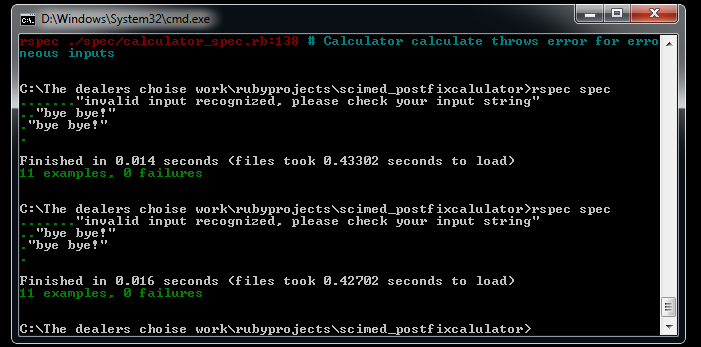Feels so good doesn’t it? Now here is where the importance of holistic testing comes into picture. We have so many tests passing and we feel we did a great job but it actually sucks. Lets write a few more tests and see whats wrong. These tests will be for successive calculations.

```it "calculates the input: test case 7" do

calculator = Calculator.new
result = calculator.calculate('6 5 2 - 4 + *')
expect(result).to eq 42.0

end
it "calculates the input: test case 8" do

calculator = Calculator.new
result = calculator.calculate('1 2 3 4 5 * * * *')
expect(result).to eq 120.0

end
it "calculates the input: test case 9" do

calculator = Calculator.new
result = calculator.calculate('1 2 + 3 * 4 + 5 *')
expect(result).to eq 65.0
end
it "calculates the input: test case 10" do

calculator = Calculator.new
result = calculator.calculate('1 2 * 3 * 4 * 5 *')
expect(result).to eq 120.0
end```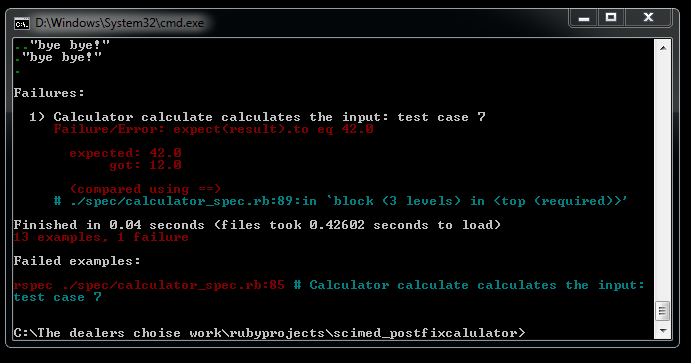Lets try and understand what went wrong for 6 5 2–4 + *

If you see the successive steps in the figure below, you will see our algorithm is right. Its operating the way it should but the only problem is we chose a wrong data structure so the operation is wrong. When we do the same operations with a stack as below, we will get the right answers. The only difference is the order of operands would be reversed.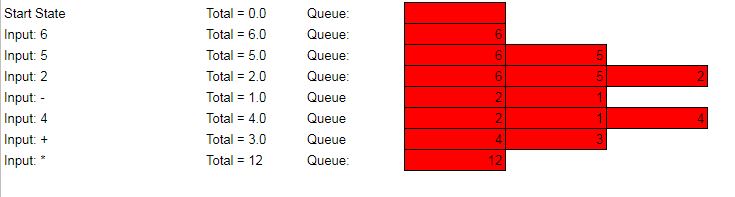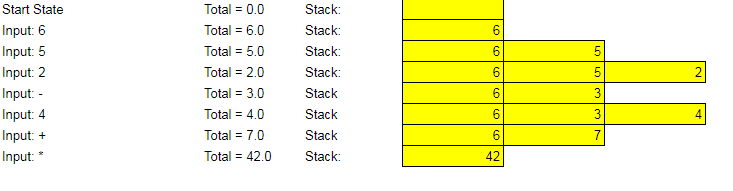Now we know the importance of writing tests holistically and spending a lot of time before moving on to actual code. Write as many tests for edge cases as possible and your life would be easy and customers would be happy. Lets try changing our code to stack:

```class Calculator
def initialize
@stack = Array.new
@total = 0.0
end
def calculate(input)
input_to_array = input.split(' ')
input_to_array.each do |i|
if i == "q" or i == "\u0004"
@stack.clear
@total = 0.0
p "bye bye!"
return
end
# input sanitization and check for legit input, if input is not legit, calculator should raise error.
if !!(i =~ /\A\d|\+|\-|\*|\/\z/)
if i == '+'
# p "addition"
@first = @stack.pop.to_f
@second = @stack.pop.to_f
@total = @first + @second
@stack << @total

elsif i == '-'
# p "subtraction"
@first = @stack.pop.to_f
@second = @stack.pop.to_f
@total =  @second - @first
@stack << @total
elsif i == '/'
# p "division"
@first = @stack.pop.to_f
@second = @stack.pop.to_f
@total = @second / @first
@stack << @total

elsif i == '*'
# p "multiplication"
@first = @stack.pop.to_f
@second = @stack.pop.to_f
@total = @first * @second
@stack << @total

else
# p i
@total = i.to_f
@stack << @total
end
else
p "invalid input recognized, please check your input string"
return "invalid input recognized"
end
end
return @total
end
end```

Running the tests again: rspec specSo now is it all good?

Nope! Now is the time for completion of the first loop. RED GREEN REFACTOR

Step 7. Refactoring

What we need?
1. More modular code
2. DRY (Don’t repeat yourself)
3. Ease of maintenance
4. Single Responsibility Principle
5. Better Design for future enhancements

Problems with previous code:

1. Repetitions of steps in each operations: Pop last two, and put it back in the stack
2. Lack of single Responsibility, each method is doing way more than what its name suggests
3. Spaghetti code, not easy to maintain and add new operations

Solution:
I won’t follow all the design strategies overall but will use few to clean my code a bit. One can have separate class for santization etc to follow SRP more closely but I chose to do it in the calculator itself anduse SRP(single responsibility principle) to divide methods and give them single responsibility. You can also chose to use proc or class method but this is one of the way you can do it.

Changes:
1. Separate method for input sanitization, so another developer can just focus on that (This can also be separated in another class but lets leave it like this for now)
2. Extract process method out to do all the processing.
3. Extract and have different methods for each operations so afterwards its easy to change a operation.

```class Calculator
def initialize
@stack = Array.new
@total = 0.0
end
def calculate(input)
input_to_array = input.split(' ')
input_to_array.each do |i|
#first check if q or CTRL+D has been entered, if so exit the program
if i == "q" or i == "\u0004"
@stack.clear
@total = 0.0
p "bye bye!"
return
end
# input sanitization and check for legit input, if input is not legit, calculator should raise error.
if sanitize(i)

if i == '+'
@total = process(@stack, method(:add), @total)
elsif i == '-'
@total = process(@stack, method(:subtract), @total)
elsif i == '/'
@total = process(@stack, method(:divide), @total)
elsif i == '*'
@total = process(@stack, method(:multiply), @total)
else
@total = i.to_f
@stack << @total
end
else
p "invalid input recognized, please check your input string and press q to restart program"
return "invalid input recognized"
end
end
return @total
end
#Below this are the method used after refactoring for more usabiity, modularity and ease of change in future directions
def sanitize(input)
#refactored, change the regular expression when adding new functionalities for santization for more inputs
if !!(input =~ /\A\d|\+|\-|\*|\/\z/)
return true
end
return false
end
#A wrapper function for processing at one place
def process(stack, operation, total)
input1 = stack.pop.to_f
input2 = stack.pop.to_f
#Reverse order for consistency as per stack LIFO
total = operation.call(input2,input1)

stack << total
return total
end
#refactored, DRY. Use .call and pass method as symbols as per operations you want to support. Add any other opeartions here and call in process
def add(input1, input2)
return (input1 + input2)
end
def subtract(input1, input2)
return (input1 - input2)
end
def divide(input1, input2)
# We could add a check like below for divide by zero but the repl program itself return infinity in such a case.
# if input1 == 0.0
#  return "Infinity"
# end
return (input1 / input2)
end

def multiply(input1, input2)
return (input1 * input2)
end
end```

lets run our tests again: rspec spec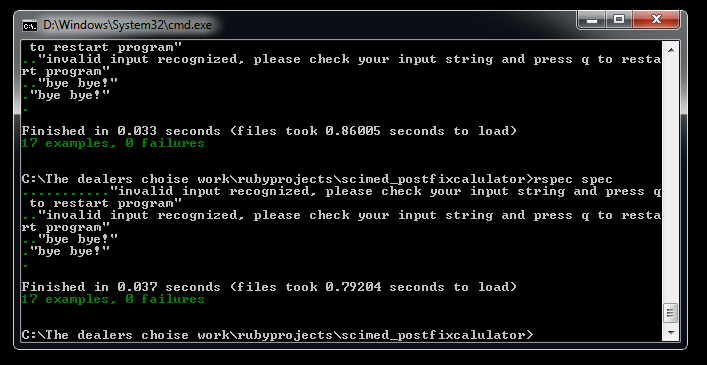Step 8. finally, lets wrap it up by writing a simple repl command line interface loop.

In the lib folder, create a new file say, runrepl.rb

```require "./calculator"
calculator = Calculator.new
print " Welcome! Please Read Instructions below: \n"
print " Press q or CTRL+C or CTRL+D on Windows OS and press enter to exit\n"
print " Press any other key and press enter to start calculator\n"
input = "#{gets}"
print "Enter your postfix expression with space in-between, press enter to calculate: \n"
while (input != "q\n") and (input != "\u0004\n")
print "input expression > "
input = "#{gets}"
print "result: #{calculator.calculate(input)} \n"
end
print "Thank you!\n"```

Go to the lib folder and run:

`ruby runrepl.rb`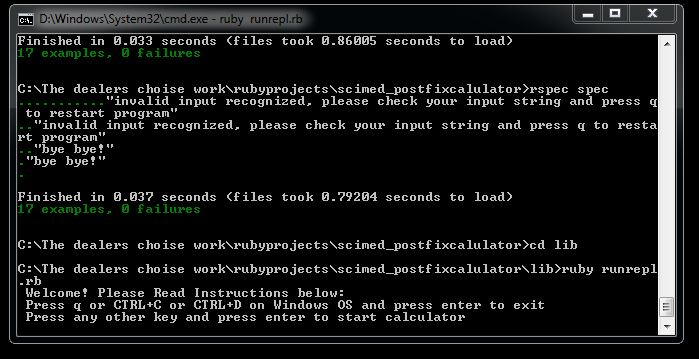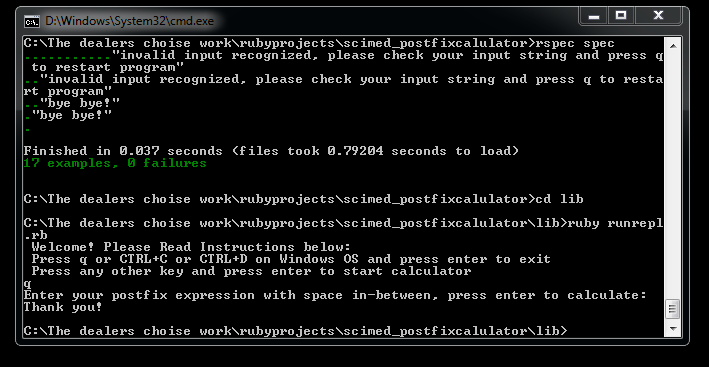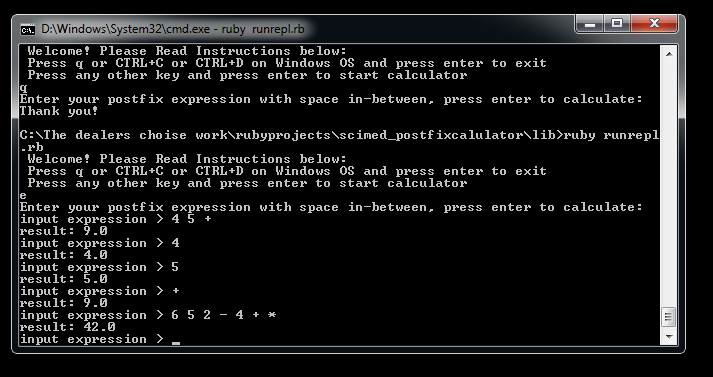There we have it. A simple college problem solving guided by test driven development and following RED GREEN REFACTORING method. This is by no means a perfect code. There can be more refactoring which could be done, more clarity and more test cases which can be written. Its just a code I wrote within time constraints. Feel free to comment or suggest if I missed on something :)

I hope it helps someone out there, esp. those in college to understand the importance of writing tests before writing code. If it does and you get in the habit of writing or even talking and thinking with Test Driven Development in each interview and each project, You gonna rock it!!

Thank you :)

Owned & Maintained by Saurav Prakash

If you like what you see, you can help me cover server costs or buy me a cup of coffee though donation :)